MATHEMATICAL INDUCTION

1. Use the method of mathematical induction to prove the following important results:

(a)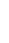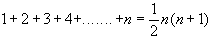(b)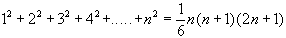(c)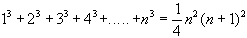(d)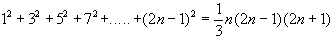2. Use the principle of mathematical induction to prove that:

(a)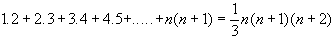(b)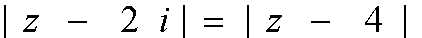3. Prove, by mathematical induction, that:

(a)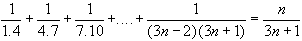(b)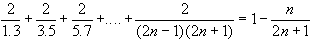4. Use the method of mathematical induction to prove that:

(a)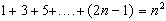(b)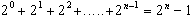5. Determine the formula for the sum to n terms, and use the method of mathematical induction to prove these formulae:

(a) 1 + 4 + 7 + 10 + . . . (b) 1 + 4 + 42 + 43 + . . .

6. Prove that:

(a)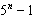is divisible by 4.

(b)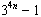is divisible by 80.

(c)is divisible by x - 1.

7. Show that:

(a)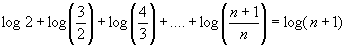(b)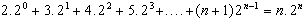8. Prove the following identities by finite (methamatical) induction for integers n > 1

(a) If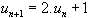and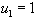then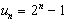.

(b)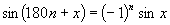[hints: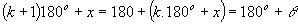, say]

9. Show by mathematical induction that:

(a)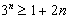(b)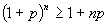where p>-1

10. Use the method of mathematical induction to prove that:

(a)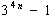is diivisible by 5. [hints: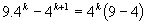]

(b)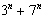is divisible by 10, if n is odd [hints: in step 2, assuming the result is true for n = k, where k is odd, then we have to show that it is true for the next odd integers (n = k+2) ].

Prove the following by using the method of mathematical induction:

11.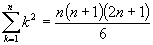12.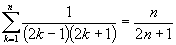13.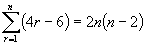14. The sum of n terms of an arithmetic sequence whose first term is a and common difference d is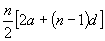15.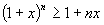for x > 0.

16.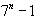is divisible by 3.

17.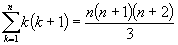18. n(n + 1) is an even number.

19. 2 + 4 + 6 + . . . . . + 2n = n(n + 1)

20.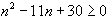for n > 1.

21.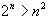for n > 4.

22. n(n + 1) + (n + 2) is divisible by 3.

23.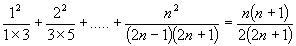24.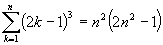25.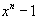is divisible by (x - 1)

26.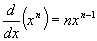for all x > 1

27.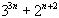is divisible by 5.

28. The sum of the cubes of three consecutive integers is divisible by 3.

29.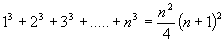30.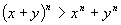31.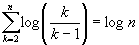32.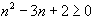for all x > 1

33.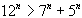for x > 2.